# ISEE Upper Level Math : How to find the length of the side of a hexagon

## Example Questions

### Example Question #1 : Hexagons

What is the sidelength, in feet and inches, of a regular hexagon with perimeter 16 feet?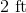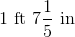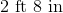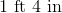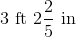Explanation:

Divide the perimeter 16 feet by 6 to get the sidelength of the hexagon in feet: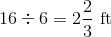Two-thirds of a foot is equal to eight inches, so this sidelength is 2 feet 8 inches.

### Example Question #2 : Hexagons

The perimeter of a regular hexagon is one mile. Give the sidelength in feet.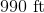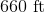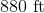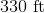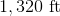Explanation:

One mile is equal to 5,280 feet, so divide this by 6: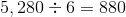feet

### Example Question #3 : Hexagons

A hexagon has a perimeter of 114in. Find the length of one side.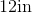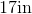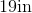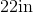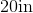Explanation:

A hexagon has 6 equal sides.  The formula to find perimeter of a hexagon is: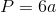where a is the length of any side.  Now, to find the length of one side, we will solve for a

We know the perimeter of the hexagon is 114in.  So, we will substitute and solve for a.  We get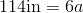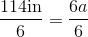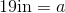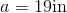Therefore, the length of one side of the hexagon is 19in.

### Example Question #4 : Hexagons

The perimeter of a hexagon is 96cm. Find the length of one side.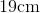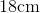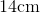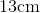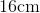Explanation:

A hexagon has 6 equal sides. To find the length of one side of a hexagon, we will use the formula to find the perimeter.where a is the length of one side. So, we will solve for a.

Now, we know the perimeter of the hexagon is 96cm. So, we get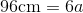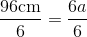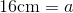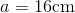Therefore, the length of one side of the hexagon is 16cm.